Java Games: Flashcards, matching, concentration, and word search.# Science 9: Basic Chemistry (Chemical formulas and reactions) for Friday 12/6

AB
The element that forms the backbone of organic molecules is ___.carbon
Two or more elements bond together to form ____.compounds
A compound which has bonds formed by sharing electrons is called a ___.molecule (technically, a molecule like oxygen which is made of two oxygen atoms is also a molecule because it is held together with covalent bonds, but it is not a compound because it does not have two or more different elements in it)
How many oxygen atoms are in H20? (The two is supposed to be a small subscript)One (there are two hydrogens)
What is the chemical formula for a molecule of glucose?.,In a chemical equation, the _____ are on the left side of the arrow.reactants
In a chemical equation, the ___ are on the right side of the arrow.products
The process of using energy from the sun to make food molecules like glucose is called ____.photosynthesis,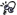What are the products of photosynthesis?,food (usually glucose) and oxygen,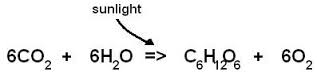What are the reactants of photosynthesis?,water and carbon dioxide,Which two molecules are needed for photosynthesis to occur?,water and carbon dioxide,What are the waste products of cellular respiration?,carbon dioxide and water,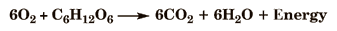,What are the reactants of cellular respiration?,oxygen and glucose,Glucose is a type of ____.sugar
A reaction that gives off energy (loses energy) is called an _____ reactionexothermic,A reaction that requires more energy than it gives off is called an ___ reaction.endothermic,Cellular respiration is an example of an ___ reaction,exothermic reaction,Burning wood is an example of an ____ reaction.exothermic (because it gives off more energy than is needed to get it started),Photosynthesis is an example of an ___ reaction.,endothermic (because it requires more energy than it gives off),How many phosphorus atoms are in the compound whose chemical formula is shown below?,One (The subscripts that come after the element's chemical symbol tell you how many atoms there are. The 3 tells you that there are 3 hydrogen atoms and the 4 tells you that there are 4 oxygen atoms. If there is no number, then it is assumed that there is one atom),How many oxygen atoms are in the compound whose chemical formula is shown below?,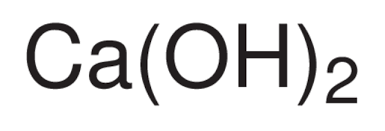Two (The subscripts that come after a chemical symbol tell you how many atoms of that element there are. In this case, since the subscript comes after a parentheses, it applies to both the oxygen and the hydrogen in parenthesis. It is written this way to show you that there are two hydroxide groups (OH) attached to the calcium atom, as shown in the picture below.),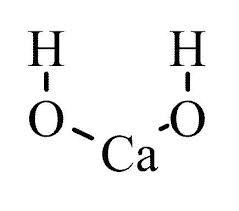A chemical equation is balanced if there are ____.The SAME NUMBER of EACH TYPE of atom on both sides of the arrow (Notice there are 6 carbon atoms, 12 hydrogen atoms, and 18 oxygen atoms both at the beginning at at the end of this reaction),What does the number 6 written in front of the carbon dioxide (CO2) tell you?,That there are six molecules of CO2,The big numbers in front of the molecules in the equation below are called _____ while the smaller numbers are called _____.,Coefficients, subscripts,The bigger numbers (coefficients) that are written in front of the molecular formulas of each molecule tell you the number of ___ located to the ____ of the coefficient.,molecules there are, right (for instance, there are six molecules of oxygen required in this reaction and 6 molecules of carbon dioxide are produced along with 6 molecules of water),The smaller numbers (subscripts) in chemical formulas tell you the number of _______ located to the ____ of the subscript.,atoms of the element, left (For instance, the 12 tells you that there are 12 hydrogen atoms in a molecule of glucose),When balancing a chemical equation, you may only change the ___, not the _____.Coefficients, subscripts (Remember that coefficients are the big numbers written in front of the molecules and subscripts are the small numbers that are written down low)This activity was created by a Quia Web subscriber. Learn more about QuiaCreate your own activities